Examples for capacitors

Example #1

Problem:

Consider two plates, separated by d=1.5 cm where the electric field between them is 100 (V/m), and the charge on the plates is 30.0 mC. What is the capacitance of the plates?

Solution:

The Capacitance is:2.0E-5 (f)

Example #2

Problem:

Consider a capacitor made of two 0.05 m2 plates separated by 0.5 mm. If the capacitance is 3.0 nf, what is the relative permeability, k, of the material between the plates?

Solution: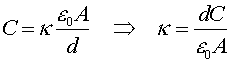3.4

Example #3

Problem: part a.)

What is the capacitance of the following segment of a circuit?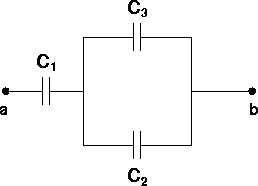DATA: C1=C2=C3=3.0 mf

Solution:

The capacitance of the two in parallel is C34=C3+C4. The capacitance of the entire circuit is: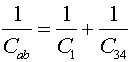2.0 (mf)

part b.)

If a voltage Vab = 6.0 V is applied, what is the charge on each capacitor?

Solution:

The charge on the first capacitor IS the charge sucked in, which can be found from the capacitance.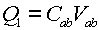There is a charge Q1 on the opposite side of the first capacitor, which must therefore have come equally from the next capacitors. Therefore, Q2=Q3=(1/2)Q1.

Q1 = 12 mC,     Q2 = Q3 = 6.0 mC

part c.)

What is the voltage across each capacitor?

Solution:

Use Vi=Qi/Ci. Check when you are done that the voltages add up to 6.0 V.

V1 = 4.0 V,     V2 = V3 = 2.0 V

Example #4

Problem:

A capacitor has a charge of 3.0 nC when the voltage across the capacitor is 12 V. What is the energy stored in the capacitor?

Solution:

The Energy is: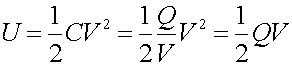12 E-9 (J)

Capacitor's index